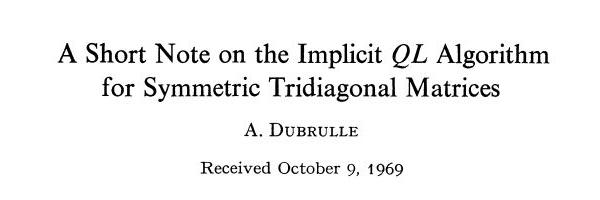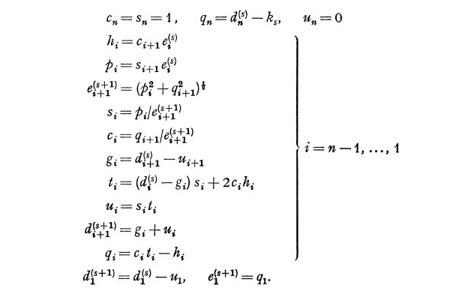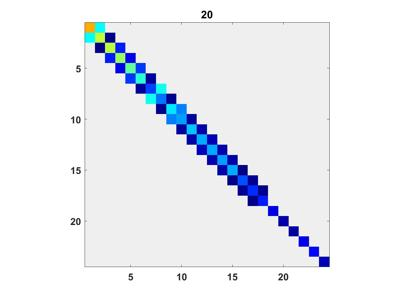# Dubrulle Creates A Faster Tridiagonal QR Algorithm

Augustin (Austin) Dubrulle deserves to be better known in the numerical linear algebra community. His version of the implicit QR algorithm for computing the eigenvalues of a symmetric tridiagonal matrix that was published in a half-page paper in Numerische Mathematik in 1970 is faster than Wilkinson's version published earlier. It is still a core algorithm in MATLAB today.

### Contents

#### QR vs. QL

"QR" and "QL" are right- and left-handed, or forward and backward, versions of the same algorithm. We are used to thinking of factoring a matrix into an orthogonal factor, Q, and an upper or right triangular factor, R. This leads to QR algorithms. But for reasons having to do with starting a loop at $1$ rather than $n-1$, the authors of the Handbook Series on Linear Algebra decided to use left triangular and QL algorithms.

#### Austin Dubrulle

Augustin (Austin) Dubrulle is the only guy that I know who improved on an algorithm of Jim Wilkinson. Austin was born in France. He began his career in the early 1960's with IBM at their Institut de Calcul Scientifique in Paris. In 1968 he transferred to an IBM center in Houston, and began work on SSP, IBM's Scientific Subroutine Package. After studying papers and books by Wilkinson and Parlett, Austin derived his own version of the implicit QR algorithm for computing the eigenvalues of a symmetric tridiagonal matrix. He was careful about the use of common subexpressions to save operations. This is especially important when computing eigenvectors because the transformations are applied to an accompanying full matrix. Austin told me that he wrote up his result in the style of the Linear Algebra series in Numerische Mathematik and asked IBM for permission to submit the paper for publication. He was told by company lawyers that, in view of the Justice Department antitrust suit, IBM could not give away software, and that the ALGOL included in the paper was software. Austin tried to make the case that ALGOL was primarily a means of human communication and secondarily a programming language of little practical use, but that argument did not fly. When Martin and Wilkinson published their implicit algorithm, Austin was encouraged to realize that his version had fewer operations in the inner loop and so would be faster. He arranged to meet Wilkinson at the 1969 University of Michigan numerical analysis summer school. Jim encouraged Austin to submit a note with just the algorithm, no provocative software, to the series. Here is a recreation of Dubrulle's half-page 1970 paper in Numerische Mathematik. This is the entire paper. You can get the original here or purchase it here. (You can get the Martin and Wilkinson contribution here or purchase it here.)

#### Dubrulle's Paper

'********************************************************************************************'The following is a formulation of the QL algorithm with implicit shift which requires fewer operations than the explicit and implicit algorithms described in  and . Let $d_i^{(s)}$ $(i=1,...,n)$ and $e_i^{(s)}$ $(i=1,...,n-1)$ be the diagonal and codiagonal elements of the matrix at the $s$ -th iteration and $k_s$ the shift. Then the $(s+1)$ -st iteration can be expressed as follows.Acknowledgements. The author wishes to thank Dr. J.H. Wilkinson for his helpful comments References 1. Bowdler, H., Martin, R.S., Reinsch, C., Wilkinson, J.H.: The QR and QL algorithms for symmetric matrices. Numerische Mathematik 11, 293-306 (1968). 2. Martin, R. S., Wilkinson, J.H.: The implicit QL algorithm. Numerische Mathematik 12, 377-383 (1968).
A. Dubrulle
IBM Corporation
Industry Development-Scientific Applications
6900 Fannin
Houston, Texas 77025, U.S.A.
'********************************************************************************************'

#### Underflow and Overflow

Dubrulle's algorithm still needs a little work. The computation of the next $e_{i+1}$ as the square root of the sum of squares is dangerous. There is potential for unnecessary underflow or overflow. I discussed this in a post almost two years ago on Pythagorean Addition. I don't suggest actually using the pythag algorithm. Instead, do something like this.
if abs(p) >= abs(q)
c = q/p;  t = sqrt(1+c^2);
e(i+1) = t*p;  s = 1/t;  c = c*s;
else
s = p/q;  t = sqrt(1+s^2);
e(i+1) = t*q;  c = 1/t;  s = s*c;
end


#### Handbook Alters History

When the papers from Numerische Mathematik were collected to form the 1971 Handbook for Automatic Computation, Volume II, Linear Algebra, almost all of them where reprinted without change. But, despite what its footnote says, Contribution II/4, The Implicit QL Algorithm, never appeared in the journal. The paper is the merger of Dubrulle's note and the Martin-Wilkinson contribution that it references. The ALGOL procedures, imtql1 and imtql2, are new. The authors of Contribution II/4 are A. Dubrulle, R.S.Martin, and J.H.Wilkinson, although the three of them never worked together. Proper credit is given, but I'm afraid that an interesting little bit of history has been lost.

#### Impact Today

Dubrulle's version of the implicit tridiagonal QR algorithm continues to be important today. We had it in EISPACK. Look at the source for IMTQL1.F. You will see code that is very similar to Austin's note. Except there is a call to a function PYTHAG to compute E(I+1). In LAPACK the imtql1 and imtql2 functions are combined into one subroutine named DSTEQR.F Here again you will see code that is very similar to Austin's note. Now PYTHAG is named DLATRG. I haven't run any timing experiments myself recently, but I have been told that the method of choice for the real symmetric matrix eigenvalue problem is Cuppen's divide and conquer algorithm, as perfected and implemented in LAPACK's DSTEDC.F. A large problem is recursively broken into smaller ones. When the matrices become small enough, and eigenvectors are desired, DSTEQR is called. This is Dubrulle's version of implicit QR. Then the recursion is unwound to produce the final result.

#### eigsvdgui

I could use an appropriate graphic for this post. Here is a picture of the tridiagonal QR algorithm in action, generated by eigsvdgui from Numerical Computing with MATLAB.Published with MATLAB® R2015a
|